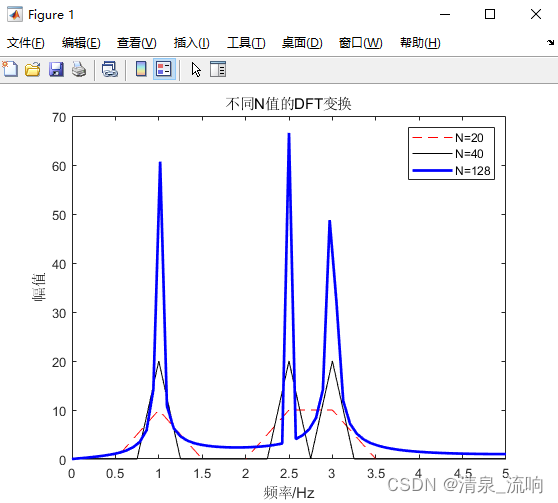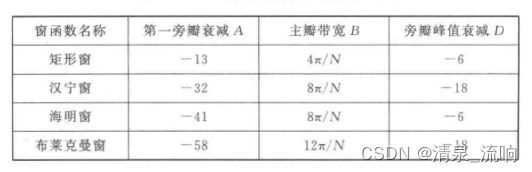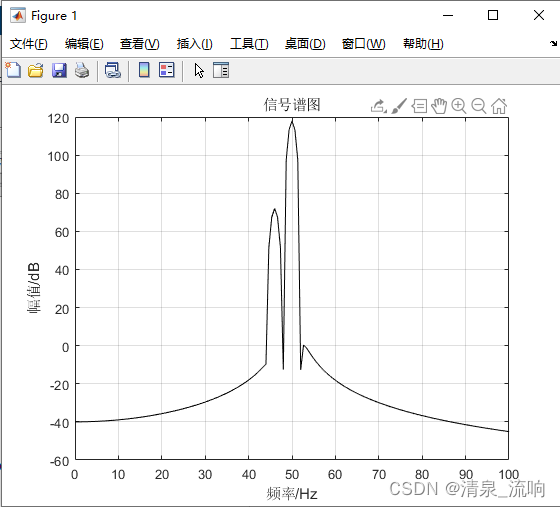# 选择信号的采样频率和信号长度的技巧

x(t)=sin(2π*f1*t)＋sin(2π*f2*t)＋sin(2π*f3*t)

N1≥[fs/△fmin]=10/2.5-1=6.6

N2≥[fs/△fmin]=10/3-2.5=20

clear all; clc; close all;

M=256; fs=10;                                   % 设置数据长度M和采样频率fs
f1=1; f2=2.5; f3=3;                             % 设置3个正弦信号的频率
t=(0:M-1)/fs;                                   % 设置时间序列
x=cos(2*pi*f1*t)+cos(2*pi*f2*t)+cos(2*pi*f3*t); % 计算出信号波形

X1=fft(x,20);                                   % FFT变换
X2=fft(x,40);
X3=fft(x,128);
freq1=(0:10)*fs/20;                              % 计算3个信号在频域的频率刻度
freq2=(0:20)*fs/40;
freq3=(0:64)*fs/128;
% 作图
plot(freq1,abs(X1(1:11)),'r--');hold on;
plot(freq2,abs(X2(1:21)),'k-');
plot(freq3,abs(X3(1:65)),'b-','linewidth',2);
legend('N=20','N=40','N=128');
title('不同N值的DFT变换');
xlabel('频率/Hz'); ylabel('幅值');
set(gcf,'color','w');①选择何种类型窗函数较合适？
②采样长度N应为多少？
③分析信号的实际频谱。

20lg|(1.55/311)*5%|=-80dBN≥fs/△fmin*(K+M)=[8000/(50-46)]*（3+3）=12000

clear all; clc; close all;

f1=50; a1=311.46;                        % 设置第1个分量的频率与幅值
f2=46; a2=1.57;                          % 设置第2个分量的频率与幅值
N=12000;                                 % 设置数据长度N
fs=8000;                                 % 设置采样频率fs
t=(0:N-1)/fs;                            % 设置时间刻度
x=a1*cos(2*pi*f1*t)+a2*cos(2*pi*f2*t);   % 设置信号
freq=(0:N/2)*fs/N;                       % 设置频率刻度
wind=blackman(N)';                       % 给出布莱克曼窗函数
X=fft(x.*wind);                          % FFT
plot(freq,20*log10(abs(X(1:N/2+1))),'k');% 作图
grid; xlim([0 100])
xlabel('频率/Hz'); ylabel('幅值/dB');
title('信号谱图');
set(gcf,'color','w');参考文献：MATLAB数字信号处理85个实用案例精讲——入门到进阶；宋知用（编著）

06-073725
11-27102
03-16784
11-08989
10-175701
06-126798
08-236107
07-23232
02-278604
01-031万+
05-077403
04-12557
11-045万+
07-131万+
02-175745
05-011303
09-17838
04-12557
10-282万+
11-25677

### “相关推荐”对你有帮助么？

•非常没帮助
•没帮助
•一般
•有帮助
•非常有帮助被折叠的  条评论 为什么被折叠?到【灌水乐园】发言¥2 ¥4 ¥6 ¥10 ¥20余额支付 (余额：-- )扫码支付获取中扫码支付点击重新获取扫码支付1.余额是钱包充值的虚拟货币，按照1:1的比例进行支付金额的抵扣。
2.余额无法直接购买下载，可以购买VIP、C币套餐、付费专栏及课程。余额充值